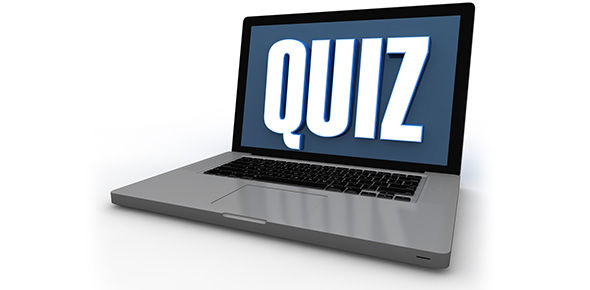# Chapter 7 Test

23 Questions | Total Attempts: 167SettingsChapter 7 Test Geometry Transformations

• 1.
What type of transformation is shown?
• A.

Rotation

• B.

Translation

• C.

Reflection

• D.

Dilation

• 2.
Which type of transformation is NOT a rigid transformation?
• A.

Rotation

• B.

Translation

• C.

Reflection

• D.

Dilation

• 3.
Which combination of transformations would result in the same image as a reflection about the y-axis?
• A.

Reflect over y-axis, rotate 180 degrees

• B.

Reflect over x-axis, rotate 90 degrees

• C.

Rotate 180 degrees, reflect over x-axis

• D.

Rotate 90 degrees, reflect over y-axis

• 4.
Which of these images cannot be achieved using a single rigid transformation?ABCD
• A.

A

• B.

B

• C.

C

• D.

D

• E.

E (don't pick this... there is no E)

• 5.
Reflect this preimage about the line y=x.Which of these is the resulting image?ABCD
• A.

A

• B.

B

• C.

C

• D.

D

• 6.
What is the component form of this vector.
• A.

• B.

• C.

• D.

• E.

-2/5 (don't pick this one, this is slope)

• 7.
What are the coordinates of the image after point (10,2010) is translated by the vector <2,-1>?
• A.

(2020,1)

• B.

(12,2009)

• C.

(12,2011)

• D.

(-2000,1)

• 8.
The point J at (12,18) is translated by (x,y) --> (x-10, y-20).  Then, the resulting point J' is reflected over the x-axis.  What are the coordinates of J''?
• A.

(2,2)

• B.

(2,-2)

• C.

(-2,2)

• D.

(-2,-2)

• 9.
The point T at (-1,4) is rotated 90o counterclockwise, then translated by <-2,-3>and finally reflected over the line y=x.  What are the coordinates of T'''?
• A.

(-4,-6)

• B.

(4,-2)

• C.

(-2,2)

• D.

(-2,-6)

• 10.
The point (6,3) is reflected over the y-axis.  What are the coordinates of the image?
• A.

(3,6)

• B.

(-3,-6)

• C.

(-6,3)

• D.

(6,-3)

• 11.
The point (6,3) is reflected over the line y=-x.  What are the coordinates of the image?
• A.

(3,6)

• B.

(-3,-6)

• C.

(-6,3)

• D.

(6,-3)

• 12.
What is the length of the segment connecting points A (-2,-1) and B (2,-4)?In other words, what is the measure of segment AB?Or, in still other words, what is distance between points A and B?These are all the same question.  What is the correct answer?
• A.

(-3,-2)

• B.

(0,-2.5)

• C.

-3/4

• D.

5

• 13.
Point Z (3,-4) is rotated 90o clockwise.  What are the coordinate of the image, Z'?
• A.

(-4,-3)

• B.

(-3,-4)

• C.

(4,3)

• D.

(3,4)

• 14.
Point H (-2,-9) is rotated 90 degrees clockwise about the origin.Now, what are the coordinate of the image, H'?
• A.

(-9,-2)

• B.

(-9,2)

• C.

(9,-2)

• D.

(2,9)

• E.

Cannot tell because it does not say if it is rotated clockwise or counterclockwise

• 15.
Which of these is NOT the same as a 180 degree rotation?
• A.

Reflect over the x-axis, then reflect over the y-axis

• B.

Reflect over the line y=x, then reflect over the line y=-x

• C.

Rotate 270 degrees clockwise, then rotate 270 degrees clockwise

• D.

Rotate 540 degrees clockwise, then rotate 270 degrees counterclockwise

• 16.
How many lines of symmetry does a square have?
• A.

1

• B.

2

• C.

3

• D.

4

• E.

It depends on the size of the square

• 17.
Which of these is NOT a possible number of lines of symmetry for a hexagon?
• A.

4

• B.

3

• C.

2

• D.

1

• E.

0

• 18.
Does this figure have rotational symmetry?  If so, state the number of degrees needed to map it onto itself.
• A.

No

• B.

45

• C.

90

• D.

180

• 19.
What is the rotational symmetry of this wheel?State the number of degrees needed to map it onto itself.
• A.

36

• B.

72

• C.

90

• D.

180

• E.

360

• 20.
Reflect this point over the line y=1.What are the coordinates of the image?
• A.

(0,-1)

• B.

(-1,2)

• C.

(-2,-1)

• D.

(2,3)

• 21.
If the image of a point A' is (3,4) following a translation of <0,7>, then what must have been the location of the pre-image point A?
• A.

(0,3)

• B.

(0,11)

• C.

(3,-3)

• D.

(3,11)

• 22.
What is the area of this shape?  (hint, use the formula sheet)
• A.

54 square units

• B.

108 square units

• C.

810 square units

• D.

1620 square units

• 23.
Find the slope of a line passing through the following points:( -3, -1) and (-9, 2)
• A.

-2

• B.

-1/2

• C.

1/2

• D.

2

• E.

Uh, oh. I got none of these.